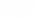## 目录

Warframe的能量主要用于驱动技能。所有Warframe都有四个技能，在基础状态下需要消耗最高100点能量使用。每一个Warframe都有自己的能量池，能够支持的技能数根据Warframe种类和具体技能的消耗量而不尽相同。对于近战导引，能量被用来提升伤害以及其他效果比如，以及驱动格挡时充能，以完全抵消伤害、反弹部分伤害给攻击者以及让敌人可被进展处决，但是每格挡一次伤害都会消耗一些能量。

## 任务中Warframe初始能量

### 能量基础值表## 能量有效值

### 对应施放性技能的有效值

$\text{能 量 有 效 值 }_{shi fang xing}=\frac{\text{能 量 上 限 }}{2-\text{技 能 效 率 }}$

$\text{能 量 有 效 值 }_{shi fang xing}\leq 4\times\text{能 量 上 限 }$

### 对应持续性技能的有效值

$\text{能 量 有 效 值 }_{chi xu xing}=\frac{\text{能 量 上 限 }\times\text{技 能 持 续 时 间 }}{2-\text{技 能 效 率 }}$

$\text{持 续 性 技 能 持 续 时 间 }=\frac{\text{能 量 有 效 值 }_{chi xu xing}}{\text{基 础 能 量 消 耗 速 率}}$

$\text{持 续 性 技 能 持 续 时 间 }=\frac{\text{能 量 上 限 }\times\text{技 能 持 续 时 间 }}{\text{基 础 能 量 消 耗 速 率 }(2-\text{技 能 效 率 })}$

$\text{持 续 性 技 能 持 续 时 间 }=\frac{\text{能 量 上 限 }}{\text{安 装 mod后 能 量 消 耗 速 率 }}$

### 例子

$\frac{50}{0.25}=200\text{ 秒 }$

$\frac{744}{0.25}=2,976\text{ 秒 }\approx 50\text{ 分 钟 }$

## 回复能量

• 能量可以通过拾起能量球获得。敌人掉落的能量球可以回复25点能量，储藏箱掉落的能量球可以回复50点能量。
• 的在击杀之后可以为其光环范围内的玩家提供不同量的能量；这取决于技能的使用时间以及敌人是被击中头部还是其他部位击杀。
• 的使得玩家可以通过站在技能目标所释放出的能量波中来回复能量（技能目标全程被击晕定身）。
• 裂隙位面可以为其内的玩家缓慢回复能量。可以与能量虹吸叠加。
• 的被动技能鼓舞可以在Octavia释放技能时为她身边的玩家持续缓慢恢复能量。
• 团队能量回复是可以回复付近队友能量的消耗品，最多400点。
• 触发熵数效果的武器可以为玩家回复其基础能量值的25%，并且能提升Warframe基础能量上限25%，持续30秒。
• 触发毁坏效果的武器可以为玩家回复其基础能量值的25%，并且能提升Warframe基础移动速度上线10%，持续30秒。
• Zenurik专精有许多帮助玩家回复能量的技能。
• 可以影响能量回复的mod：
• 可以影响能量回复的附能：

5.0
2人评价